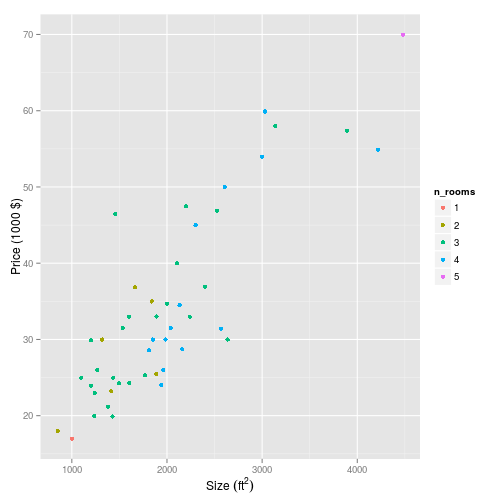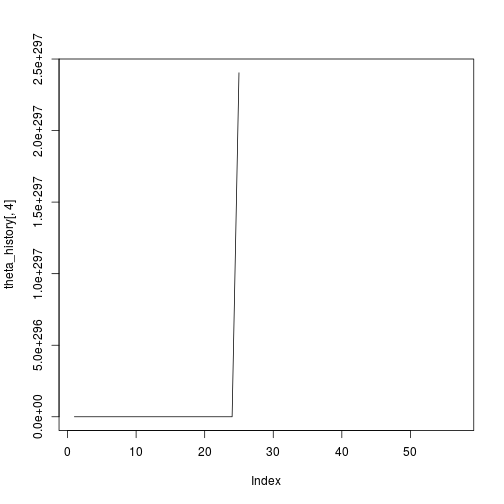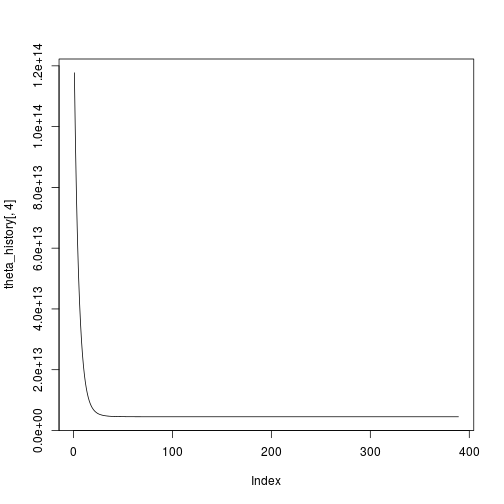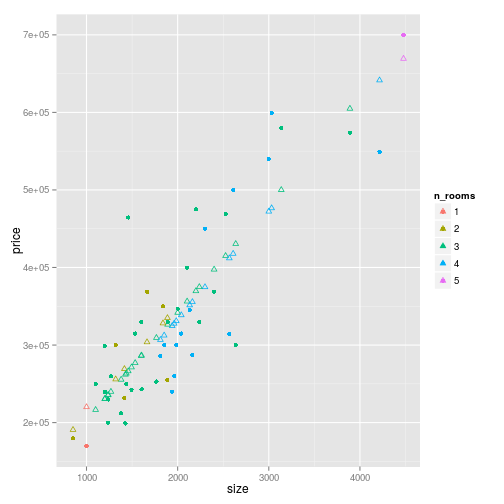### Matt Upson

Yo no soy marinero

# Multivariate linear regression

So this time I’m going to implement gradient descent for multivariate linear regression, but also using feature scaling. I’m using teh dataset provided in the machine learning course, which describes the cost of houses based on two parameters: the size in square feet, and the number of rooms, and giving prices in dollars.

First I’ll load the data and take a look at it.

So we have two $x$’s: size and n_rooms

Let’s also plot it out of interest:### Feature normalisation/scaling

To copy the exercise document:

• Subtract the mean value of each feature from the dataset.
• After subtracting the mean, additionally scale (divide) the feature values by their respective “standard deviations.”

and in the file featureNormalize.m provided with the course material, we get:

First, for each feature dimension, compute the mean of the feature and subtract it from the dataset, storing the mean value in mu. Next, compute the standard deviation of each feature and divide each feature by it’s standard deviation, storing the standard deviation in sigma.

Note that X is a matrix where each column is a feature and each row is an example. You need to perform the normalization separately for each feature.

I’ll have a go at implementing that in R.

Ok so let’s try this on our features in the housing dataset.

We can have a look to see what this has done to our values. Originally the ranges for the features were:

and

…so quite a difference.

After feature scaling these ranges are:

and

…so now much closer.

In the multivariate case, the cost function can also be written in the vectorised form:

$J(\theta)=\frac{1}{2m}(X\theta-\vec{y})^T(X\theta-\vec{y})$ Where: $X=\begin{bmatrix} (x^{(1)})^T \\ (x^{(2)})^T \\ (x^{(3)})^T \\ \vdots \\ (x^{(m)})^T \end{bmatrix}\vec{y}=\begin{bmatrix} y^{(1)} \\ y^{(2)}\\ y^{(3)} \\ \vdots \\ y^{(m)} \end{bmatrix}$

Here I use the grad() gradient descent function I defined in my post about linear regression with gradient descent.

First set up the inputs:

And simply apply the function, but on the raw data without feature scaling.

Hmm ok so that didn’t seem to work. Just out of interest, let’s plot the history:Definitely something not working there. Ok so now I’ll try it with feature scaling.

And to plot it:Great, convergence after 389 iterations. All seems well, but I want to compare this with a multiple linear regression the traditional way:

The parameters don’t match, but this is because we have scaled the features. The output from the two models will be the same. Here I check by combining the two predictions into the house_prices dataframe, and comparing them with identical().

Ok not identical, how come?

So they differ by a pretty small amount. Try the comparison more sensibly:

And now let’s plot the actual data with predictions from the multiple regression.Pretty close to a single regression model, but you can see that there are slightly different slopes for each number of rooms.